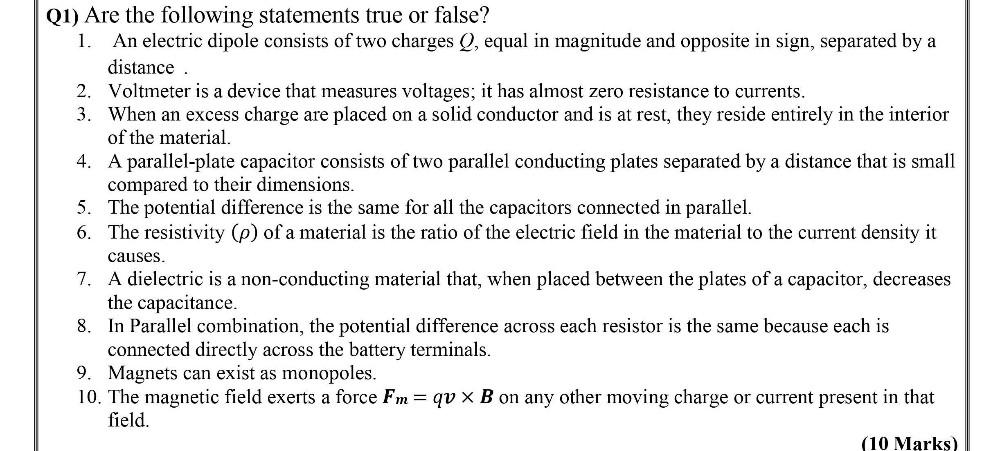Question:

# Are the following statements true or false? 1. An electric dipole consists of two charges Q, equal in magnitude and opposite in sign, separated by a distance. 2. Voltmeter is a device that measures voAre the following statements true or false? 1. An electric dipole consists of two charges Q, equal in magnitude and opposite in sign, separated by a distance. 2. Voltmeter is a device that measures voltages; it has almost zero resistance to currents. 3. When an excess charge are placed on a solid conductor and is at rest, they reside entirely in the interior of the material. 4.A parallel-plate capacitor consists of two parallel conducting plates separated by a distance that is small compared to their dimensions. 5. The potential difference is the same for all the capacitors connected in parallel. 6. The resistivity (ρ) of a material is the ratio of the electric field in the material to the current density it causes. 7. A dielectric is a non-conducting material that, when placed between the plates of a capacitor, decreases the capacitance. 8.In Parallel combination, the potential difference across each resistor is the same because each is connected directly across the battery terminals. 9. Magnets can exist as monopoles. 10. The magnetic field exerts a force Fm = qv x B on any other moving charge or current present in that field.